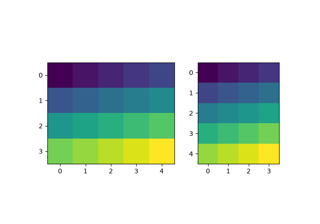# mpl_toolkits.axes_grid1.axes_divider.HBoxDivider#

class mpl_toolkits.axes_grid1.axes_divider.HBoxDivider(fig, *args, horizontal=None, vertical=None, aspect=None, anchor='C')[source]#

Bases: `SubplotDivider`

A `SubplotDivider` for laying out axes horizontally, while ensuring that they have equal heights.

Examples

Parameters:
fig`Figure`
*argstuple (nrows, ncols, index) or int

The array of subplots in the figure has dimensions ```(nrows, ncols)```, and index is the index of the subplot being created. index starts at 1 in the upper left corner and increases to the right.

If nrows, ncols, and index are all single digit numbers, then args can be passed as a single 3-digit number (e.g. 234 for (2, 3, 4)).

horizontallist of `axes_size`, optional

Sizes for horizontal division.

verticallist of `axes_size`, optional

Sizes for vertical division.

aspectbool, optional

Whether overall rectangular area is reduced so that the relative part of the horizontal and vertical scales have the same scale.

anchor(float, float) or {'C', 'SW', 'S', 'SE', 'E', 'NE', 'N', 'NW', 'W'}, default: 'C'

Placement of the reduced rectangle, when aspect is True.

new_locator(nx, nx1=None)[source]#

Create an axes locator callable for the specified cell.

Parameters:
nx, nx1int

Integers specifying the column-position of the cell. When nx1 is None, a single nx-th column is specified. Otherwise, location of columns spanning between nx to nx1 (but excluding nx1-th column) is specified.

## Examples using `mpl_toolkits.axes_grid1.axes_divider.HBoxDivider`#HBoxDivider and VBoxDivider demo

HBoxDivider and VBoxDivider demo